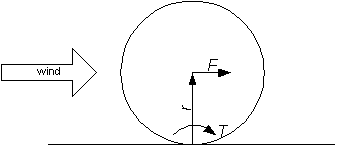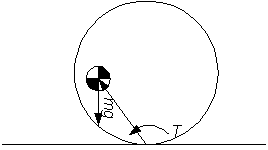Wind Force on Balls

When contemplating spherical robots that might operate outdoors, the force of the wind blowing on the ball is of interest. A detailed calculation would be quite complex, because of the interaction of the ground surface. However, for estimation purposes, we can probably bound the force.First order approach

A simple approach is to consider the force as the aerodynamic drag on a sphere acting at the center of the sphere. The drag of a sphere is actually somewhat complex for low speed flow because of boundary layer issues (why golf balls have dimples, for instance). Some web references are : http://aerodyn.org/Drag/speed-drag.html#sphere or http://www.princeton.edu/~asmits/Bicycle_web/blunt.html. We can assume as a sort of worst case that Cd=1.0 (typical for a sphere at lowish Reynolds numbers (ca. 100)).

Drag = rho * Cd * v^2 * Area

where

rho = density of air
v = velocity
Area = flat plate projected area = pi*r^2 for a sphere

In practice, the equation is rendered as K*v^2*r^2, where the constant K absorbs: air density, unit conversion factors, and pi

For v in m/sec, r in meters, and drag in Newtons, K = 1.184 kg/m^3 * 3.14159 = 3.71964

For v in mi/hr, r in feet, and drag in pounds, K = K(SI units) * .447 m/sec/(mi/hr) * 1/3.28^2 (m^2/ft^2) * 1/4.54 pounds/newton = 3.40E-2 (or approximately 1/29.4)

Some typical numbers for a 1 meter diameter sphere are given in the following table. Consider that the thing we are really concerned about is the moment which is trying to turn the sphere, so one should really divide these numbers by 2, to get the moment in Nmeter. The table shows the mass that would have to be at the upwind point of the sphere to resist this moment. Clearly, one can't put all the mass there, so one would need more mass, with the CG somewhat inside the shell.

 V (m/sec) F (Newton) Mass (kg) 1 0.93 0.047 2 3.7 0.190 5 23 1.2 10 93 4.7 15 210 11 20 370 19Second order approach

A very handy reference by Blevins (Applied Fluid Dynamics Handbook, Krieger Publishing Company, Malabar, Florida, 1992 ) gives some approximations for Cd and Cl of a cylinder near a wall (p318) at Re= 20,000. No mention is made if the incident flow is laminar or turbulent. Blevins is citing Roshko, A., et al., "Flow Forces on a Cylinder Near a Wall or Near Another Cylinder," Second National Conference on Wind Engineering Research, Colorado State University, Ft. Collins, Colorado, June, 1975.

 spacing/diameter Cd Cl 0 0.8 0.6 0.25 1.1 0.25 0.5 1.2 0.15 1.0 1.3 0.05 1.5 1.2 0.02 2.0 1.2 0 4.0 1.2 0 6.0 1.2 0

The table on page 335 in Blevins gives some numbers for a cube above a surface, and while there is a net downforce (i.e. Cl is negative), the Cd numbers appear reasonably similar. The Cd numbers appear to be of the same general magnitude as used in the first order computation.

robot/windball.htm - 13 Dec 2001 - Jim Lux